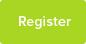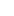# Tutor Hunt Questions

I would like to find data from the electrolysis of dilute copper sulphate with products and percentages.

3 years ago

Chemistry Question asked by Jayne

Please enter your response to the question below. The student will get a notification as soon your response has been approved by our moderation team.

You will require this equation and also percentage of which product you require.
Cu2+(aq) + 2e- ==> Cu(s) and 4OH-(aq) - 4e- ==> 2H2O(l) + O2(g
From the equation we know

* It takes a transfer of 2 moles of electrons to form 1 mole of solid copper (63.5g) from 1 mole of copper(II) ions, Cu2+

* and a transfer of 4 moles of electrons to form 1 mole of oxygen from 4 moles of hydroxide, OH- ions.

You need to know the atomic weight elements .Cu=63.5O

You also need to know basic fact of electrolysis.Mole fact
*(+) anode and (-) cathode electrode product ratio
If you know how much of a substance is made at one electrode, you can theoretically calculate the amount of substance formed at the other electrode.
Using basic math to find percentage you can work out your

:)
Answered by Zeba | 3 years ago